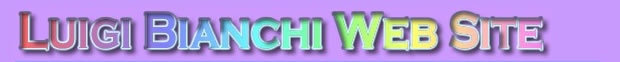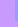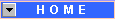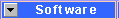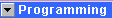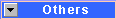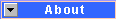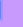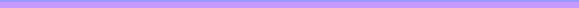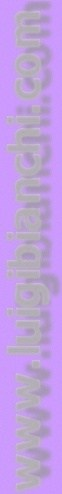# C/C++ (Programming)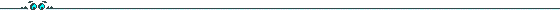Title Description Release Smart Randomizer Suppose that you want to generate N random numbers but that you want a determined number of replications for each of them: for example you want 10 times the number 1, 25 times the number 2 and 15 times the number 3. Using the std::random() function does not guarantee you that after 50 extractions you have the desired number of replications for each number. My solution is to provide a class, which does that: class RandomListInt {    public:       RandomListInt();       Init(const std::vector& items_);       int GetNext();       unsigned int Size() const;    private:       std::list > RndList; ... // some more stuff }; The Init member function receives a std::vector which represents the number of replications that one wants to generate for each element of the vector. In our case 10, 25 and 15. Its body is: RandomListInt::Init(const std::vector& items) {    RndList.clear();    for (int i = 0; i < items.size(); i++)       for (int c = 0; c < items[i]; c++)          RndList.push_back(std::make_pair(i, random(std::numeric_limits::max())));    RndList.sort(lower_rnd); } For each of the 3 elements a number of items equal to the number that they represents are stored in a std::list with associated a randomly generated number through a std::pair. Then, the list is sorted depending on the associated random number. Calls to the GetNext() member will return the index of the vector passed to the Init(...) function and remove the pair from the list. int RandomListInt::GetNext() {    int val = RndList.front().first;    RndList.pop_front();    return val; } When the RndList is empty (that is when Size() returns 0), the randomization is completed. An example of its use is the following one, where GetEventsCount() and events are members of a SimpleProtocol class of mine, and rnd_list is an instance of RandomListInt: if (!bInitialized) {    std::vectorvv_;    vv_.reserve(GetEventsCount());    for (unsigned int i = 0; i < GetEventsCount(); i++)       vv_.push_back(events[i]->GetTrialsCount());    rnd_list.Init(vv_); } if (rnd_list.Size())    return rnd_list.GetNext(); else    ..// randomization completed! 25 June 2001 HW Port IO in Win95/98 It is not true that you need a VXD to read/write from hardware port in Windows95/98. Here you can find the required code (tested with Borland C++) that I have found some years ago in a newsgroup. 27 June 2000 SmartLocale I often use locales for text formatting, but it happens quite often that I have to read/write floating-point data from a text file. What happens if, for example, an Italian user like me sets the decimal separator in a way that is unexpected to the scanf routine? One possible solution is to assume that all I/O routines should handle English formatted text. In this case, anyway, it is necessary to set/reset locales very often, at least whenever an fscanf (or fprintf) routine is called. This, obviously, happens also to all the other locales such as date, etc. For this reason I've written a small class (SmartLocale) that handles these situations: whenever you create an instance of that class, you change the local settings, and when the destructor is called, the default Windows locales are restored. For example, if I have the Italian locales (that uses the decimal separator ',') and I execute the following code, ```//current locale, Italian char  str; FILE *fin = fopen("etd128.pos", "rt"); if (fin) {  printf(str, "1) 10 / 3 = %f\n", 10. / 3.);    TSmartLocale engl(); // current locale English, I can safely read // floating point English text from file ....    printf(str, "2) 10 / 3 = %f\n", 10. / 3.);    fclose(fin); } // now back to Italian locale: engl out of scope // and destructor called printf(str, "3) 10 / 3 = %f\n", 10. / 3.);  ``` The output will be: ```1) 10 / 3 = 3,333333 2) 10 / 3 = 3.333333 3) 10 / 3 = 3,333333 ``` Note that just one line of code (the red one) was required to handle this situation. 11 June 2000